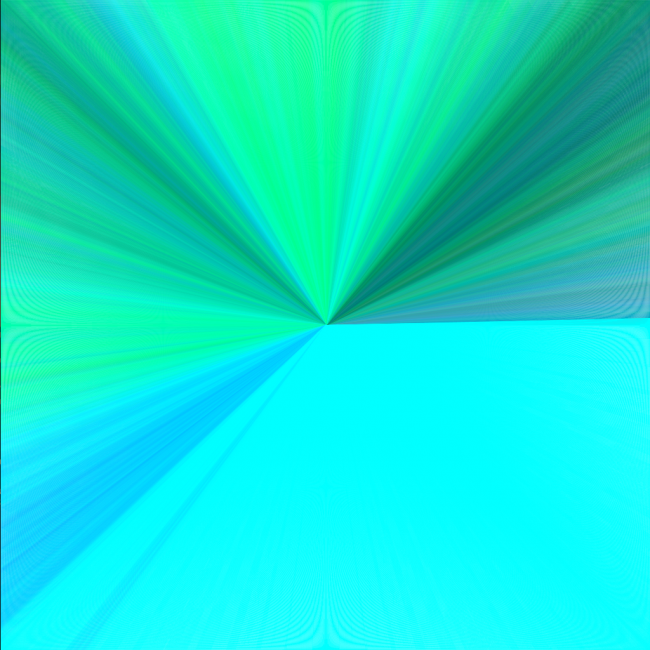#### Howdy, Stranger!

We are about to switch to a new forum software. Until then we have removed the registration on this forum.

# How to remove odd white lines/glare

edited January 2017

I just started working with Processing, and my program is working like I want to, except that there are odd white, curved lines that look almost like a glare that shouldn't be there on it. They appear on the corners and the edges, as shown here:(sorry this one isn't as pretty as it could be, gotta make it less random, also it's most easily seen on the right edge)

And here's the code (Java):

``````float lerpFrequency;
float darkestStart;
float lightestStart;
float spacing;
int x;
int y;
int eighth;
float rR;
float rG;
float rB;

void setup() {
size (1024, 1024);
background (255, 255, 255);
stroke(0);
lerpFrequency = 7;
darkestStart = 0;
lightestStart = 255;
spacing = 1;
x = width;
y = width/2;
eighth = 1;
rR = int(random(darkestStart,lightestStart));
rG = int(random(darkestStart,lightestStart));
rB = int(random(darkestStart,lightestStart));
}

void draw() {
rR = random(rR - lerpFrequency, rR + lerpFrequency);
rG = random(rG - lerpFrequency, rG + lerpFrequency);
rB = random(rB - lerpFrequency, rB + lerpFrequency);
stroke (rR, rG, rB);
//fill(rB,rG,rR); EPILEPSY WARNING

if (eighth == 1 || eighth == 8) {
y-=spacing;

if (eighth == 1 && y <= 0) {
eighth++;
} else if (eighth == 8 && y <= width/2) {
eighth = 1;
}
} else if (eighth == 2 || eighth == 3) {
x-=spacing;

if (eighth == 2 && x <= width/2 || eighth == 3 && x <= 0) {
eighth++;
}
} else if (eighth == 4 || eighth == 5) {
y+=spacing;

if (eighth == 4 && y >= width/2 || eighth == 5 && y >= width) {
eighth++;
}
} else if (eighth == 6 || eighth == 7) {
x+=spacing;

if (eighth == 6 && x >= width/2 || eighth == 7 && x >= width) {
eighth++;
}
}
//background(255);
ellipse(width/2, width/2, x, y);
}

void mouseClicked() {
setup();
}
``````

Anyway I am clueless as to why it would create these white lines, since I would assume that any overlapping pixels would just replace each other instead of leaving some white artifacts or just a rendering error from my computer, I don't know.

Tagged:

• By default, some smoothing is being applied to lines that are drawn. This is to avoid the issue of lines looking too pixelated, but it's detrimental for your sketch.

Simply add `noSmooth();` to your setup() function.

• I just tried adding noSmooth(); to the setup() function, and tested it in multiple places, and it had no effect on the image produced.

• It did here.

Also, that code doesn't match the picture.

• It doesn't? Keep in mind it's different every time, but it's significantly different? Let me try it with the code I pasted here..

• Never mind, I used the code that I posted (Accidentally posted with Ellipse() instead of Line() being drawn as a test), and added the noSmooth() to it and it worked perfectly. Thank you, and sorry for my slight incompetence :)